# Generating Trees and Other Interesting Shapes With L-SystemsIn this post, I will explore the concept of Lindenmayer systems (L-systems for short), and use them in a small demo, to generate pretty fractals.

## What Are L-Systems?

L-systems are a formalism for generating a special kind of character strings. When interpreted graphically in a certain way, these strings may represent various interesting-looking structures, including plants or trees.

First, let's introduce a couple of handy definitions. I promise to keep it short, and we'll start getting all fancy with pictures and interactive demos in the next sections.

There are several kinds of L-systems with different levels of expressiveness. We'll start with the simplest of them, called "DOL-systems". A DOL-system is defined as a tuple <A, P, L>, where:

• A is a set of symbols, which we'll refer to as the alphabet.
• P is a set of pairs (n, w), where:
• n is a character from the alphabet;
• w is any string composed from the symbols of the alphabet.
These pairs are called productions, or rules. For clarity, we'll write productions as n → w , and we'll refer to n as the predecessor, and w as the successor. Two productions are allowed to coexist in the set P only as long as their predecessors are different.
• L is a special character from the alphabet, referred to as the axiom. L must be the predecessor in one of the productions from P.

Strings are generated from the L-system by means of iterative rewriting. The initial value of your string S is equal to the axiom, L. You then apply the following operation as many times as you wish: for each symbol c in the string S, if P contains a production of the form c → w, replace c with w.

For example, let's say you have the alphabet {P, Q, a, b, c}. Your productions are: {P →abQ, Q →cPQ}. P is your axiom.

We start with P, since that's our axiom. Now, let's apply the rewriting rule. For clarity, I will highlight the symbols that are getting replaced, and the strings they are getting replaced with, using matching colors:

• PabQ (using the first production)
• abQ ⇒ abcPQ (using the second production)
• abcPQ ⇒ abcabQcPQ (using the first and second productions)
...and so forth, as many times as you wish.

For readers familiar with formal language theory, it would be helpful to note the similarity between L-systems and formal grammars at this point - the main difference is that nonterminal symbols in a string get replaced all at once.

## Interpreting L-Systems

Generating huge strings of repeating symbols seems boring, but if we attach certain graphical meaning to these symbols, we can get some interesting results. A common way of interpretation is using the generated strings as "programs" for a turtle graphics system. Let's demonstrate on a simple example.

Our alphabet shall be: {L, r, l, f, A, B}, with the axiom being L. These symbols serve as commands for a turtle graphics system:

• r causes the turtle to turn right by δ degrees.
• l causes the turtle to turn left by δ degrees.
• f, A and B all cause the turtle to move in the direction it's facing by a fixed amount n, leaving a trail behind

The following interactive demo shows a few interesting patterns obtained by using different sets of rules and varying the parameters δ and n. The alphabet and its interpretation remain fixed. You can edit the rules yourself to see what you can come up with.

The above demo uses HTML5 Canvas API to for drawing. The implementation is naive and straighforward, so I won't bore you with code snippets. If you're interested, you can view the source - I've left comments.

## Growing a Tree

Fractal curves are cool and all, but I promised there would be trees. In order to get there, we will need to make a few adjustments to the simple model that we used in the previous section.

### Branching

One thing notably missing from our demo so far is any ability to easily generate branching structures. To address this, we shall add two new symbols to the alphabet: [ and ]. Their interpretation in terms of the turtle graphics model shall be as follows:

• [ causes the current state of the turtle (position and orientation) to be pushed onto a stack
• ] pops position and orientation from the top of the stack and sets them as current.
This allows us to branch off into a substructure at any point, and then easily return to the state prior to the branch.

Below is one example of using branching structures:Branching structure generated using a single rule L→f[rL]lL and a delta angle of 30 degrees

Bonus: if you change the angle to 60 and apply enough iterations on the L-system from the example above, a nice honeycomb pattern emerges: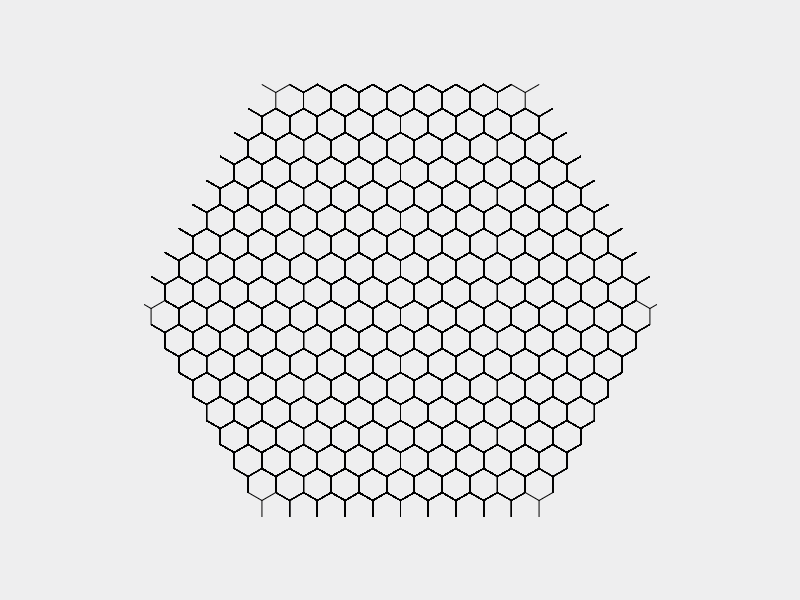### Parametric L-Systems

In the sections above, we have referred to "parameters", such as the angle δ and segment length, n. In those examples, they remain the same during each step of L-system interpretation.

A more advanced class of L-systems, called parametric OL-systems, extends this concept. In parametric OL-systems, each character of the alphabet may have an associated set of parameters. Each occurrence of the character must have an associated set of concrete parameter values. Productions are augmented with rules that both affect and depend on values of the character parameters. We'll refer to an instance of a character together with its associated parameter values as a module, and we'll represent modules as c(p1, p2, ...), where c is the character, and p1, p2, etc. are the parameter values.

To illustrate this explanation, let's consider the following example.

The alphabet is {L(g), r, l, f(n)}. Note that some of the characters have parameters associated with them now: the charater L has the parameter g (we'll refer to it as "generation"), and the character f has the parameter n (we'll refer to it as "step length"). There is only one rule, and it is L(g)→[rf(2-g/2)L(g+1)][lf(2-g/2)L(g+1)]. I know this looks kind of hairy, but the meaning will become obvious shortly. Finally, the axiom is L(0) (yes, it is important to note that in parametric OL-systems the axiom can be a module, not just a single character).

The graphical interpretation for the symbols is as follows:

• r means "turn right by 90 degrees";
• l means "turn left by 90 degrees";
• f(n) means "move forward by n units";
• L(g) is a no-op.
Hopefully, by now the meaning of the only production is more obvious : each new rewrite operation "spawns" a new generation of characters, and the higher the generation number, the shorter the step length becomes (in fact, it becomes shorter by a factor of √2 with each new generation).

If we actually visualize this L-system according to our rules, we will see that it produces a structure which may be familiar to some - an H tree.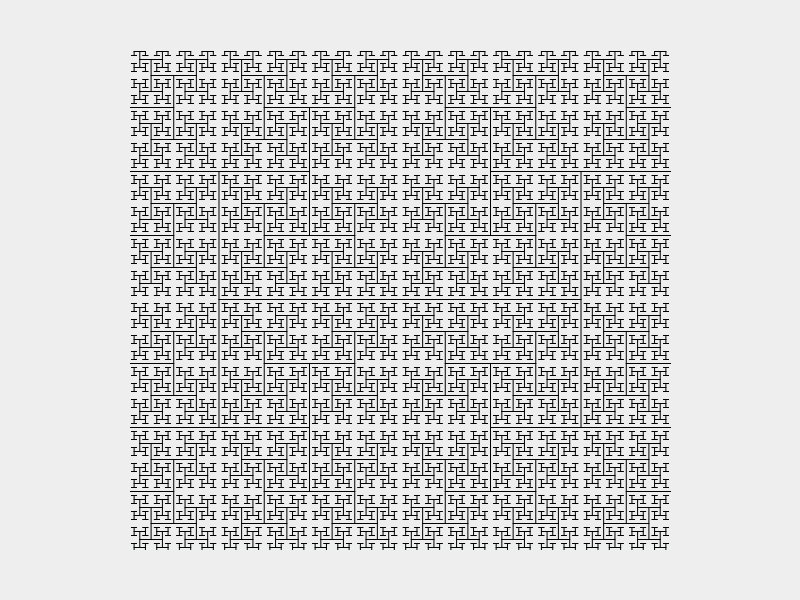The H tree.

### Randomization

Parametric OL-systems are quite powerful. However, all the images that we've seen thus far have been very artificial-looking. Nature is often irregular and kind of randomly shaped, so we'll try to liven our L-systems up a little bit by slightly varying the parameters (such as segment length and delta angle) by a random amount.

### Putting It All Together

Once we combine the three techniques described above - branching, varying parameters and randomization, we can get some interesting results.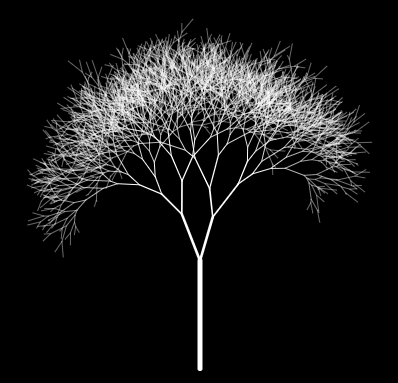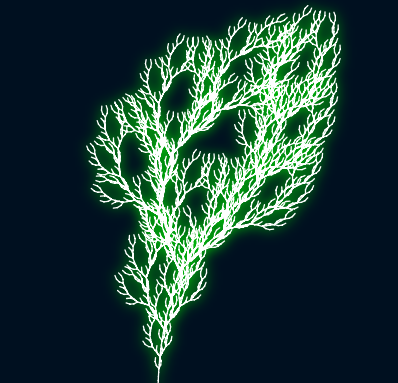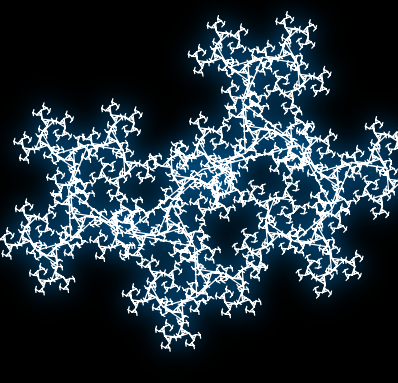The above images are generated with a small parser for parametric L-systems that I've made. I've taken a couple of shortcuts with it.

The first one is, I'm not parsing the rule notation manually: instead, I'm using PEG.js, which is a parser generator tool somewhat similar to Bison. PEG grammar for the rule notation can be found here.

The second shortcut is, I'm relying on Javascript's eval for evaluating math expressions - this is why all the expressions need to be in quotation marks, as you'll see below.

Finally, I chose to use HTML5 canvas APIs purely because of development time considerations. However, rendering performance is not that great because of that, especially for stuff that requires varying line width. I'm pretty sure I could have written a much faster renderer with WebGL, but it would have taken me more time.

## Demo Time

Let's break down the first preset here to see what's going on.

``` L(g)-> fwd("randbetween(100/(g+1)-10, 100/(g+1)+10)", "5/(g+1)") rot(defer("(3/(g+1))*Math.cos(ctx.f/60.0)")) [rot("randbetween(-20-5, -20+5)")L("g+1")] rot(defer("(3/(g+1))*Math.cos(ctx.f/70.0)")) [rot("randbetween(20-5, 20+5)")L("g+1")] rot(defer("(3/(g+1))*Math.sin(ctx.f/100.0)")); ```

Let's look at this line-by-line.

• `L(g)->` - the rules are specified in the form `symbol(param1_name, param2_name, ...) -> successor;`. Note the semicolon at the end.
• `fwd("randbetween(100/(g+1)-10, 100/(g+1)+10)", "5/(g+1)")`
• "fwd" is the symbol name. For the sake of readability, the parser allows symbol names to be more than one letter long
• "fwd" moves the turtle in the direction it's facing. The first parameter specifies how far to advance. The second one specifies line thinkness.
• Parameter values can be arbitrary Javascript expressions. They need to be included in quotation marks. They may reference any of the predecessor symbol's parameters (such as gh in this case).
• randbetween is a conveniece function returning a random floating point number in the specified range.
• `rot(defer("(3/(g+1))*Math.cos(ctx.f/60.0)"))`
• "rot" rotates the turtle by a given amount in degrees.
• "defer" is a special construct. Normally, the parameters are evaluated at the time when the string is being generated. When you "defer" an expression, however, it is evaluated at draw time rather than at string generation time. In addition to predecessor's parameters, deferred expressions can also access a special deferred context object ("ctx"), which currently has only one member, "f" - the number of the current frame. Deferred expressions are used here to add animation.
• `[rot("randbetween(-20-5, -20+5)")L("g+1")]` - this branches out slightly to the left and adds a subtree.
The remaining 3 lines are similar to what was already discussed.

The demo has a few presets that you can experiment with (though I have to warn you, error reporting on that parses is not that great). If you come up with something cool, please let me know :-)

## Further Reading

L-systems were developed in the sixties by Astrid Lindenmayer, a biologist, for studying the growth of simple multicellular organisms and algae, and later were extended for modelling more complex plants. I have only scraped the surface of this fascinating topic in this post.

If you are interested, I highly suggest you read Algorithmic Beauty of Plants for a much more rigorous and in-depth coverage of this subject.

Like this post? Follow this blog on Twitter for more!